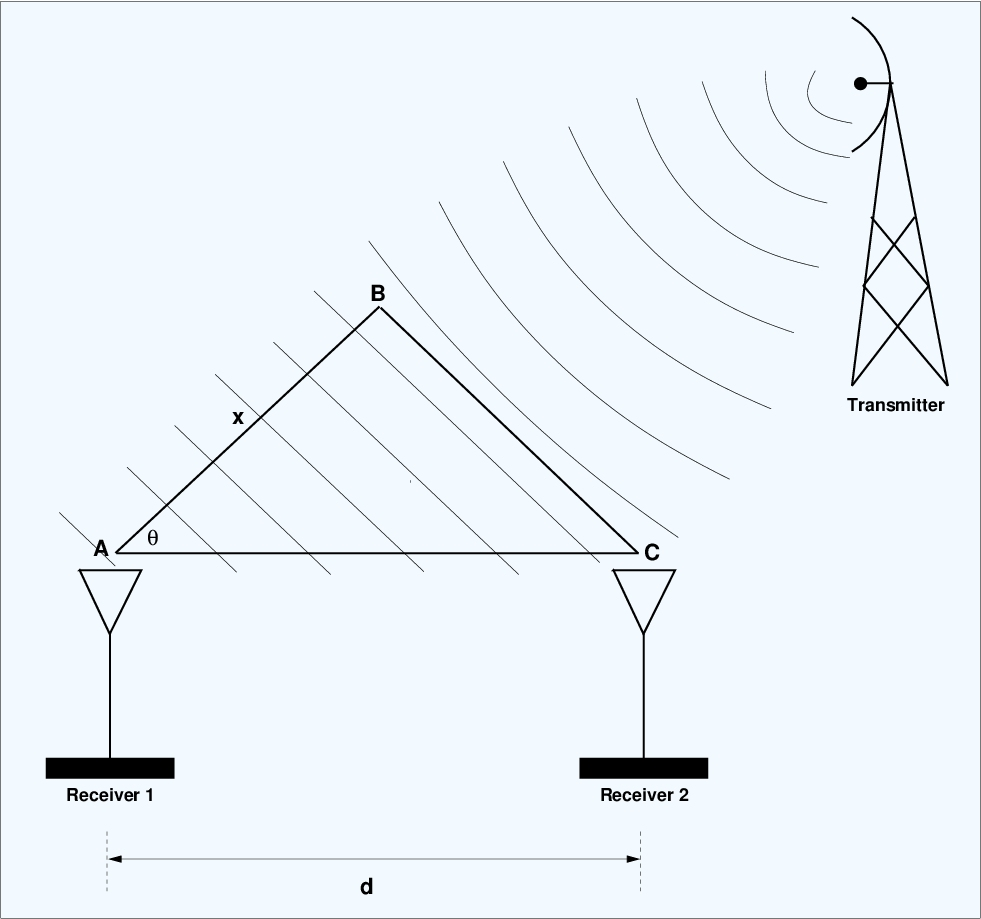## For the Beginner at CSP

Here is a list of links to CSP Blog posts that I think are suitable for a beginner: read them in the order given.

How to Obtain Help from the CSP Blog

Introduction to CSP

How to Create a Simple Cyclostationary Signal: Rectangular-Pulse BPSK

The Cyclic Autocorrelation Function

The Spectral Correlation Function

The Cyclic Autocorrelation for BPSK

Modulation recognition is one thing, holistic radio-frequency scene analysis is quite another.

So why do I obsess over cyclostationary signals and cyclostationary signal processing? What’s the big deal, in the end? In this post I discuss my view of the ultimate use of cyclostationary signal processing (CSP): Radio-Frequency Scene Analysis (RFSA). Eventually, I hope to create a kind of Star Trek Tricorder for RFSA.

## CSP-Based Time-Difference-of-Arrival Estimation

Time-delay estimation can be used to determine the angle-of-arrival of a signal impinging on two spatially separated signals. This estimation problem gets hard when there is cochannel interference present.

Let’s discuss an application of cyclostationary signal processing (CSP): time-delay estimation. The idea is that sampled data is available from two antennas (sensors), and there is a common signal component in each data set. The signal component in one data set is the time-delayed or time-advanced version of the component in the other set. This can happen when a plane-wave radio frequency (RF) signal propagates and impinges on the two antennas. In such a case, the RF signal arrives at the sensors with a time difference proportional to the distance between the sensors along the direction of propagation, and so the time-delay estimation is also commonly referred to as time-difference-of-arrival (TDOA) estimation.Figure 1. Illustration of the geometric relationship between a transmitter and two receivers in the context of time-delay estimation (or time-difference-of-arrival estimation).

Consider the diagram shown in Figure 1. A distant transmitter emits a signal that is well-modeled as a plane wave once it reaches our two receivers. An individual wavefront of the signal arrives at the two sensors at different times.

The line segment AB is perpendicular to the direction of propagation for the RF signal. The angle$\theta$ is called the angle of arrival (AOA). If we could estimate the AOA, we can tell the direction from which the signal arrives, which could be useful in a variety of settings. Since the triangle ABC is a right triangle, we have$\displaystyle \cos (\theta) = \frac{x}{d}. \hfill (1)$

When$\theta = 0$, the wavefronts first strike receiver 2, then must propagate over$x=d$ meters before striking receiver 1. On the other hand, when$\theta = 90^\circ$, each wavefront strikes the two receivers simultaneously. In the former case, the TDOA is maximum, and in the latter it is zero. The TDOA can be negative too, so that$180^\circ$ azimuthal degrees can be determined by estimating the TDOA.

In general, the wavefront must traverse$x$ meters between striking receiver 2 and striking receiver 1,$\displaystyle x = d \cos(\theta). \hfill (2)$

Assuming the speed of propagation is$c$ meters/sec, the TDOA is given by$\displaystyle D = \frac{x}{c} = \frac{d\cos{\theta}}{c} \mbox{\rm \ \ seconds}. \hfill (3)$

In this post I’ll review several methods of TDOA estimation, some of which employ CSP and some of which do not. We’ll see some of the advantages and disadvantages of the various classes of methods through inspection, simulation, and application to captured data. Consider this post as a starting point to a study or development effort rather than as a definitive performance characterization.

## Signal Selectivity

We can estimate the spectral correlation function of one signal in the presence of another with complete temporal and spectral overlap provided the signal has a unique cycle frequency.

In this post I describe and illustrate the most important property of cyclostationary statistics: signal selectivity. The idea is that the cyclostationary parameters for a single signal can be estimated for that signal even when it is corrupted by strong noise and cochannel interferers. ‘Cochannel’ means that the interferer occupies a frequency band that partially or completely overlaps the frequency band for the signal of interest.

A mixture of received RF signals, whether cochannel or not, is accurately modeled by the simple sum of the signals, as in$x(t) = s_1(t) + s_2(t) + \ldots + s_K(t) + w(t), \hfill (1)$

where$w(t)$ is additive noise. We can write this more compactly as$x(t) = \displaystyle \sum_{k=1}^K s_k(t) + w(t). \hfill (2)$